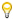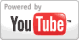The Quadratic Formula - Algebra Lesson
Grade: 7 - 12 | In this lesson, students learn to solve quadratic equations in the form ax^2 + bx + c = 0 using the quadratic formula. Students also learn to derive the quadratic formula using completing the square in the first example problem in this lesson.Add to My DashboardAlgebra
For Other Topics :Quiz Games by TopicInteractive DiagramsFlow Chart GamesVocabulary GamesSlide PuzzlesJigsaw PuzzlesCreate PresentationsBrain GamesVideos by Topic### Search Videos & Games